Visitors Online: 0 | Monday 09th December 2019CBSE Guess > Papers > Important Questions > Class XII > 2009 > Chemistry > Chemistry By Mr. Vivek Yadav CBSE CLASS XII
Q. 1. The unit of equivalent conductance is: Q. 2. Give the relationship between free energy change and EMF of a cell. Q. 3. How much amount of a substance is deposited by 1 coulomb? Q. 4. Write the relationship between molar conductivity and specific conductivity. Q. 5. What is the reference electrode in determining the standard electrode potential? Q. 6. Write the use of platinum foil in the hydrogen electrode. Q. 7. How will you identify whether the given electrolyte is a strong or a weak? Q. 8. Name the metal that can be used in cathodic protection of iron against rusting. Q. 9. Which cells were used in the Apollo space program? Q. 10. What are the units of molar conductivity? Q. 11. Why it is necessary to use a salt bridge in a galvanic cell? Q. 12. Define-(i) Faraday’s constant (ii) Electrochemical equivalent Q. 13. What is corrosion? Q. 14. What is Galvanisation ? Q. 15. Define Kohlrausch’s law. Q. 16. When 3 ampere of electricity is passed for 45 minutes 2.0 g of metal is deposited. Find equivalent weight of metal. Q. 17. Find the value of equilibrium constant from the following data-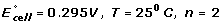Q. 18. Calculate standard free energy change for the following chemical reaction –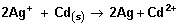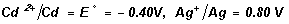Q. 19. Represent the following cell reactions as galvanic cell–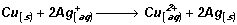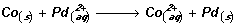Q. 20. Define and give one example of each of the following- Primary Cells Secondary Cell Fuel Cell Q. 21. What is Nernst equation? Write its expression for single electrode and cell. Q. 22. Find the emf of following cell -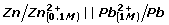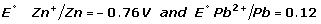Q. 23. Predict if the following reaction is feasible or not , and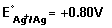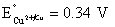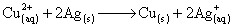Paper By Mr. Vivek Yadav